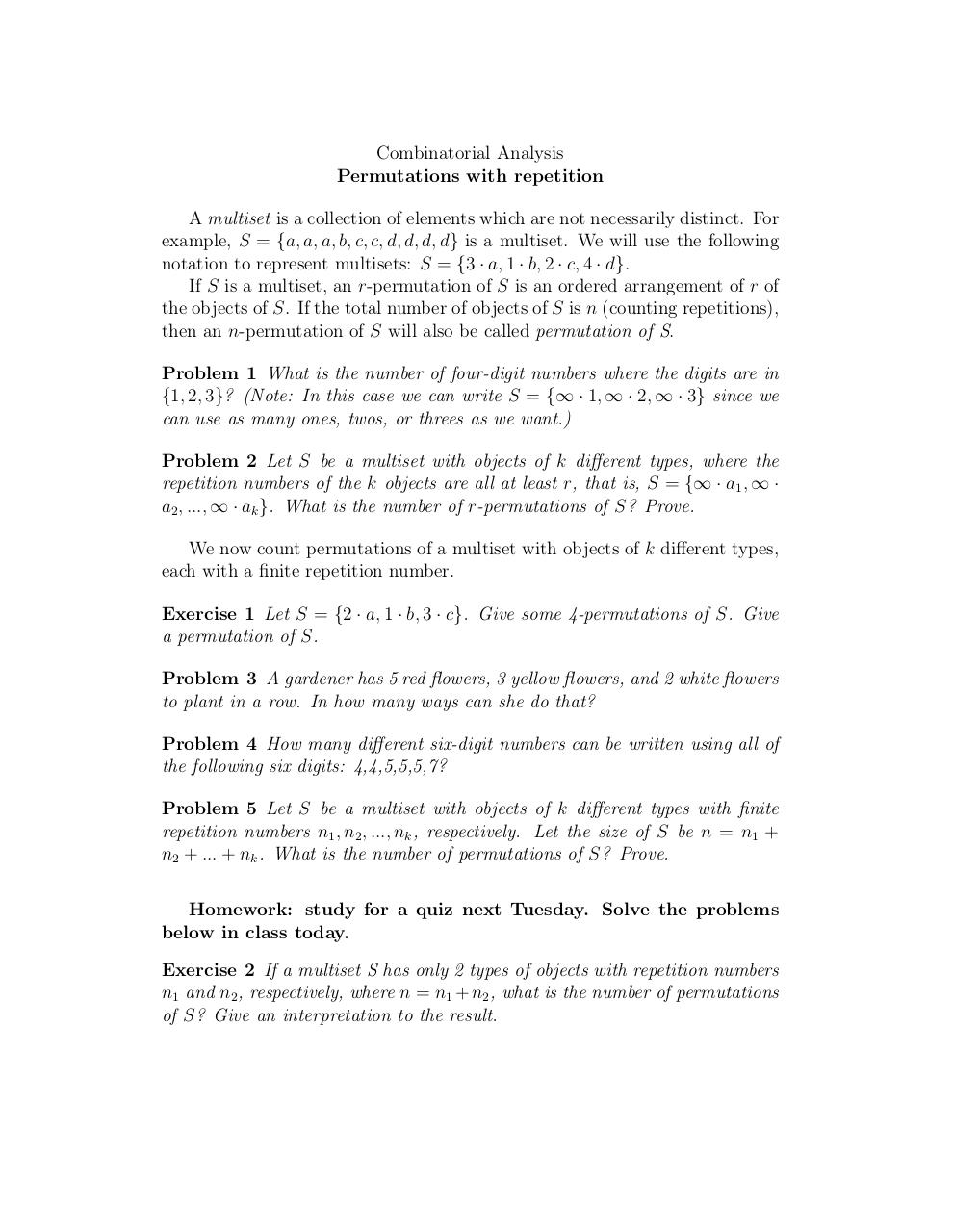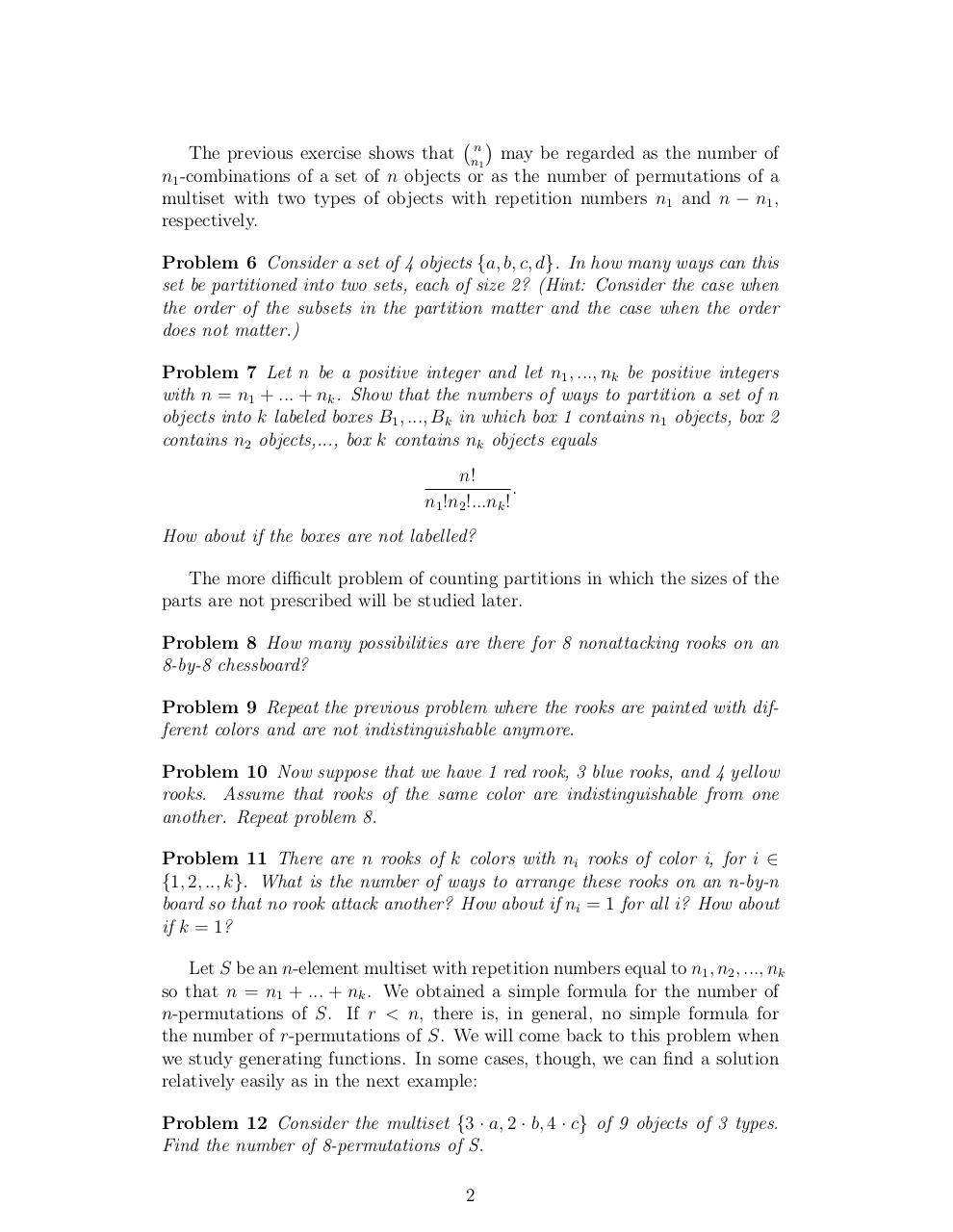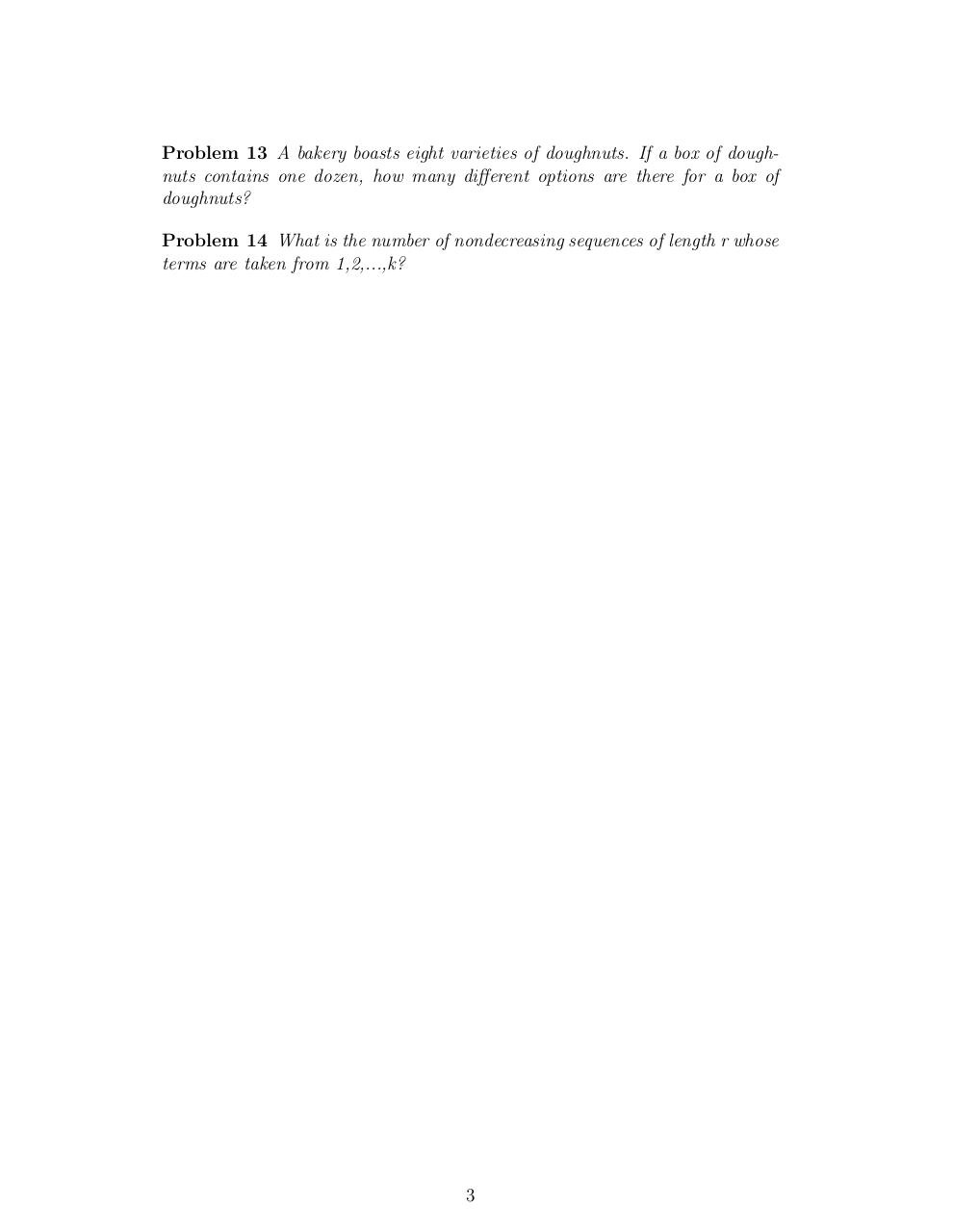# Day 8 Permutations with repetition .pdf

### File information

Original filename: Day 8-Permutations with repetition.pdf

This PDF 1.4 document has been generated by TeX / pdfTeX-1.40.3, and has been sent on pdf-archive.com on 24/01/2014 at 06:06, from IP address 169.231.x.x. The current document download page has been viewed 1018 times.
File size: 104 KB (3 pages).
Privacy: public file

Day 8-Permutations with repetition.pdf (PDF, 104 KB)

### Document preview

Combinatorial Analysis
Permutations with repetition
A multiset is a collection of elements which are not necessarily distinct. For
example, S = {a, a, a, b, c, c, d, d, d, d} is a multiset. We will use the following
notation to represent multisets: S = {3 · a, 1 · b, 2 · c, 4 · d}.
If S is a multiset, an r-permutation of S is an ordered arrangement of r of
the objects of S. If the total number of objects of S is n (counting repetitions),
then an n-permutation of S will also be called permutation of S.
Problem 1 What is the number of four-digit numbers where the digits are in
{1, 2, 3}? (Note: In this case we can write S = {∞ · 1, ∞ · 2, ∞ · 3} since we
can use as many ones, twos, or threes as we want.)
Problem 2 Let S be a multiset with objects of k different types, where the
repetition numbers of the k objects are all at least r, that is, S = {∞ · a1 , ∞ ·
a2 , ..., ∞ · ak }. What is the number of r-permutations of S? Prove.
We now count permutations of a multiset with objects of k different types,
each with a finite repetition number.
Exercise 1 Let S = {2 · a, 1 · b, 3 · c}. Give some 4-permutations of S. Give
a permutation of S.
Problem 3 A gardener has 5 red flowers, 3 yellow flowers, and 2 white flowers
to plant in a row. In how many ways can she do that?
Problem 4 How many different six-digit numbers can be written using all of
the following six digits: 4,4,5,5,5,7?
Problem 5 Let S be a multiset with objects of k different types with finite
repetition numbers n1 , n2 , ..., nk , respectively. Let the size of S be n = n1 +
n2 + ... + nk . What is the number of permutations of S? Prove.
Homework: study for a quiz next Tuesday. Solve the problems
below in class today.
Exercise 2 If a multiset S has only 2 types of objects with repetition numbers
n1 and n2 , respectively, where n = n1 + n2 , what is the number of permutations
of S? Give an interpretation to the result.


The previous exercise shows that nn1 may be regarded as the number of
n1 -combinations of a set of n objects or as the number of permutations of a
multiset with two types of objects with repetition numbers n1 and n − n1 ,
respectively.
Problem 6 Consider a set of 4 objects {a, b, c, d}. In how many ways can this
set be partitioned into two sets, each of size 2? (Hint: Consider the case when
the order of the subsets in the partition matter and the case when the order
does not matter.)
Problem 7 Let n be a positive integer and let n1 , ..., nk be positive integers
with n = n1 + ... + nk . Show that the numbers of ways to partition a set of n
objects into k labeled boxes B1 , ..., Bk in which box 1 contains n1 objects, box 2
contains n2 objects,..., box k contains nk objects equals
n!
.
n1 !n2 !...nk !
How about if the boxes are not labelled?
The more difficult problem of counting partitions in which the sizes of the
parts are not prescribed will be studied later.
Problem 8 How many possibilities are there for 8 nonattacking rooks on an
8-by-8 chessboard?
Problem 9 Repeat the previous problem where the rooks are painted with different colors and are not indistinguishable anymore.
Problem 10 Now suppose that we have 1 red rook, 3 blue rooks, and 4 yellow
rooks. Assume that rooks of the same color are indistinguishable from one
another. Repeat problem 8.
Problem 11 There are n rooks of k colors with ni rooks of color i, for i ∈
{1, 2, .., k}. What is the number of ways to arrange these rooks on an n-by-n
board so that no rook attack another? How about if ni = 1 for all i? How about
if k = 1?
Let S be an n-element multiset with repetition numbers equal to n1 , n2 , ..., nk
so that n = n1 + ... + nk . We obtained a simple formula for the number of
n-permutations of S. If r &lt; n, there is, in general, no simple formula for
the number of r-permutations of S. We will come back to this problem when
we study generating functions. In some cases, though, we can find a solution
relatively easily as in the next example:
Problem 12 Consider the multiset {3 · a, 2 · b, 4 · c} of 9 objects of 3 types.
Find the number of 8-permutations of S.
2

Problem 13 A bakery boasts eight varieties of doughnuts. If a box of doughnuts contains one dozen, how many different options are there for a box of
doughnuts?
Problem 14 What is the number of nondecreasing sequences of length r whose
terms are taken from 1,2,...,k?

3#### HTML Code

Copy the following HTML code to share your document on a Website or Blog

#### QR Code### Related keywords